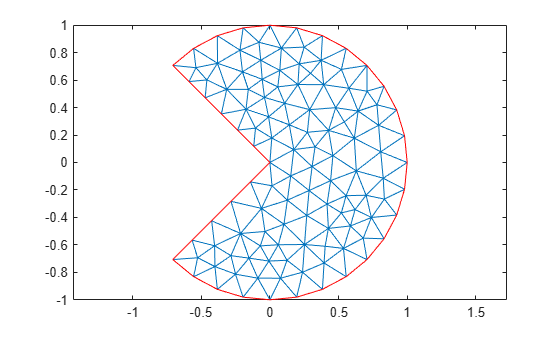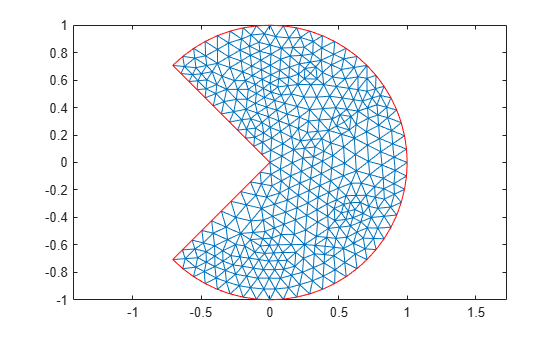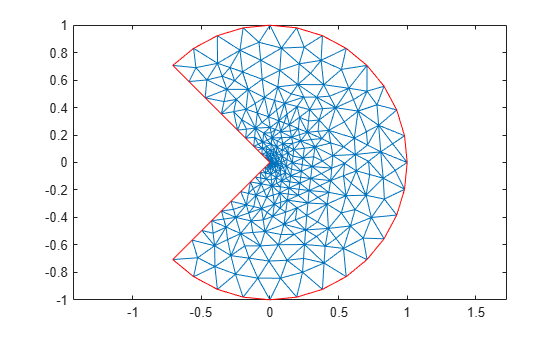# pdejmps

(Not recommended) Error estimates for adaptation

`pdejmps` is not recommended. Use meshes represented as `FEMesh` objects instead of `[p,e,t]` meshes. For more information, see Compatibility Considerations.

## Syntax

``errf = pdejmps(p,t,c,a,f,u,alpha,beta,m)``

## Description

example

````errf = pdejmps(p,t,c,a,f,u,alpha,beta,m)` calculates the error indication function used for mesh adaptation. The columns of `errf` correspond to triangles, and the rows correspond to the equations in the PDE system.The function computes the error indicator E(K) for each triangle K as$E\left(K\right)=\alpha {‖{h}^{m}\left(f-au\right)‖}_{K}+\beta {\left(\frac{1}{2}\sum _{\tau \in \partial K}{h}_{\tau }^{2m}{\left[{n}_{\tau }\cdot \text{\hspace{0.17em}}\left(c\nabla {u}_{h}\right)\right]}^{2}\right)}^{1/2}$where ${n}_{\tau }$ is the unit normal of edge $\tau$ and the braced term is the jump in flux across the element edge. Here, α and β are weight indices, and m is an order parameter. The norm is an L2 norm computed over the element K.```

## Examples

collapse all

Solve the Laplace equation over a circle sector, with Dirichlet boundary conditions u = cos(2/3atan2(y,x)) along the arc and u = 0 along the straight lines. Use the original coarser mesh and the refined mesh, and calculate the error indication function in both cases.

Generate and plot a mesh for the circle sector geometry.

```[p,e,t] = initmesh('cirsg'); pdemesh(p,e,t)```Solve the Laplace equation.

`u = assempde('cirsb',p,e,t,1,0,0);`

Calculate the error indication function for each mesh triangle. Use the weight indices $\alpha =0.15$, $\beta =0.15$, and the order parameter m = 1.

```alpha = 0.15; beta = 0.15; m = 1; errf = pdejmps(p,t,1,0,0,u,alpha,beta,m);```

Find the maximum value of the error indication function.

`max(abs(errf))`
```ans = 0.0306 ```

Refine the original mesh and plot the result.

```[p,e,t] = refinemesh('cirsg',p,e,t); pdemesh(p,e,t)```Solve the same equation on the refined mesh, and calculate the error indication function for each mesh triangle. Use the same values for the weight indices and the order parameter.

```u = assempde('cirsb',p,e,t,1,0,0); errf = pdejmps(p,t,1,0,0,u,alpha,beta,m);```

Find the maximum value of the error indication function.

`max(abs(errf))`
```ans = 0.0194 ```

Solve the same equation using the `adaptmesh` function.

`[u,p,e,t] = adaptmesh('cirsg','cirsb',1,0,0);`
```Number of triangles: 197 Number of triangles: 201 Number of triangles: 216 Number of triangles: 233 Number of triangles: 254 Number of triangles: 265 Number of triangles: 313 Number of triangles: 344 Number of triangles: 417 Number of triangles: 475 Number of triangles: 629 Maximum number of refinement passes obtained. ```

Plot the mesh.

`pdemesh(p,e,t)`Calculate the error indication function for each mesh triangle.

`errf = pdejmps(p,t,1,0,0,u,alpha,beta,m);`

Find the maximum value of the error indication function.

`max(abs(errf))`
```ans = 0.0024 ```

## Input Arguments

collapse all

Mesh node points, specified as a 2-by-`Np` matrix of points (nodes), where `Np` is the number of nodes in the mesh. For details on mesh data representation, see `initmesh`.

Data Types: `double`

Mesh elements, specified as a `4`-by-`Nt` matrix of triangles, where `Nt` is the number of triangles in the mesh. For details on mesh data representation, see `initmesh`.

Data Types: `double`

PDE coefficient, specified as a scalar, matrix, character vector, character array, string scalar, string vector, or coefficient function. `c` represents the c coefficient in the scalar PDE

`$-\nabla \cdot \left(c\nabla u\right)+au=f$`

or in the system of PDEs

`$-\nabla \cdot \left(c\otimes \nabla u\right)+au=f$`

The coefficients `c`, `a`, and `f` can depend on the solution `u` if you use the nonlinear solver by setting the value of `'Nonlin'` to `'on'`. The coefficients cannot be functions of the time `t`.

Example: `'cosh(x+y.^2)'`

Data Types: `double` | `char` | `string` | `function_handle`

PDE coefficient, specified as a scalar, matrix, character vector, character array, string scalar, string vector, or coefficient function. `a` represents the a coefficient in the scalar PDE

`$-\nabla \cdot \left(c\nabla u\right)+au=f$`

or in the system of PDEs

`$-\nabla \cdot \left(c\otimes \nabla u\right)+au=f$`

The coefficients `c`, `a`, and `f` can depend on the solution `u` if you use the nonlinear solver by setting the value of `'Nonlin'` to `'on'`. The coefficients cannot be functions of the time `t`.

Example: `2*eye(3)`

Data Types: `double` | `char` | `string` | `function_handle`

PDE coefficient, specified as a scalar, matrix, character vector, character array, string scalar, string vector, or coefficient function. `f` represents the f coefficient in the scalar PDE

`$-\nabla \cdot \left(c\nabla u\right)+au=f$`

or in the system of PDEs

`$-\nabla \cdot \left(c\otimes \nabla u\right)+au=f$`

The coefficients `c`, `a`, and `f` can depend on the solution `u` if you use the nonlinear solver by setting the value of `'Nonlin'` to `'on'`. The coefficients cannot be functions of the time `t`.

Example: `char('sin(x)';'cos(y)';'tan(z)')`

Data Types: `double` | `char` | `string` | `function_handle`

PDE solution, specified as a vector.

• If the PDE is scalar, meaning that it has only one equation, then `u` is a column vector representing the solution u at each node in the mesh.

• If the PDE is a system of N > 1 equations, then `u` is a column vector with `N*Np` elements, where `Np` is the number of nodes in the mesh. The first `Np` elements of `u` represent the solution of equation 1, the next `Np` elements represent the solution of equation 2, and so on.

Weight index, specified as number.

Data Types: `double`

Weight index, specified as a number.

Data Types: `double`

Order parameter, specified as number.

Data Types: `double`

## Output Arguments

collapse all

Error indicator, returned as a matrix with the number of columns equal to the number of triangles `t` and the number of rows equal to the number of PDEs in the system.

• Each matrix row corresponds to an equation in the PDE system.

• Each column corresponds to a triangle.

## Version History

Introduced before R2006a

expand all